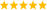# LabVIEW

cancel
Showing results for
Did you mean:

Highlighted

## How can i find the intersection in the given figureMessage 1 of 8
(513 Views)
Highlighted

## Re: How can i find the intersection in the given figure

Hi Rajesh,

I guess you want to find the point [1, 2] as "intersection".

From what you have shown:

1. Create an array of points (aka cluster of X and Y value) from your two input arrays.
2. Test for any duplicate points in your point array (use Search1DArray…)

(When you want to calculate points where lines cross: this is simple mathematics you should have learned in school. Apply math…)

Best regards,
GerdWusing LV2011SP1 + LV2017 (+LV2019 sometimes) on Win10+cRIO
Message 2 of 8
(477 Views)
Highlighted

## Re: How can i find the intersection in the given figure

Your intersection is actually at one of the actual points and thus is a set of coordinates that occurs twice. Given this information, the solution us trivial. (In addition, the start and end points overlap to close the curve, so keep that in mind)

Programming is typically more interesting and should work with any finite set of arbitrary line segments. As a first step, generate two random lines defined by two points each and see if they cross (or touch), and if so where. Solve the general problem!LabVIEW Champion. It all comes together inGCentralWhat does "Engineering Redefined" mean??
Message 3 of 8
(465 Views)
Highlighted

## Re: How can i find the intersection in the given figure

Thanks, GerdW

But I have some doubts

Here it is clearly visible that where the line intersects so you suggest search for the intersect element. But if a graph is complicated with 10000 data-set then this might not work as it may be difficult to locate the point exactly

Suppose I need to calculate this intersection with online data then what I can do.

Thank you again.

Message 4 of 8
(435 Views)
Highlighted

## Re: How can i find the intersection in the given figure

Hi Rajesh,

@Rajesh10 wrote:

Suppose I need to calculate this intersection with online data then what I can do.

Apply basic math!

Best regards,
GerdWusing LV2011SP1 + LV2017 (+LV2019 sometimes) on Win10+cRIO
Message 5 of 8
(428 Views)
Highlighted

Message 6 of 8
(425 Views)
Highlighted

## Re: How can I find the intersection in the given figure

@Rajesh10 wrote:

No it isn't.  It is a bunch of line segments between a series of points that are defined by an X array and Y array.

What you have is not really a LabVIEW problem.  At least not yet.  It is a Math/Geometry problem.

How would you find the answer if all you had was pencil and paper?

Message 7 of 8
(418 Views)
Highlighted

## Re: How can i find the intersection in the given figure

Once you solve the problem I gave you earlier, make it into a subVI, then go inspect every line segment in order and see if it touches any line segment you already looked at. You also need to deal with special cases (e.g. two lines overlap, two segments are identical, one point touched another line but the lines do not cross, one long line crosses several other segments, etc.).LabVIEW Champion. It all comes together inGCentralWhat does "Engineering Redefined" mean??
Message 8 of 8
(377 Views)